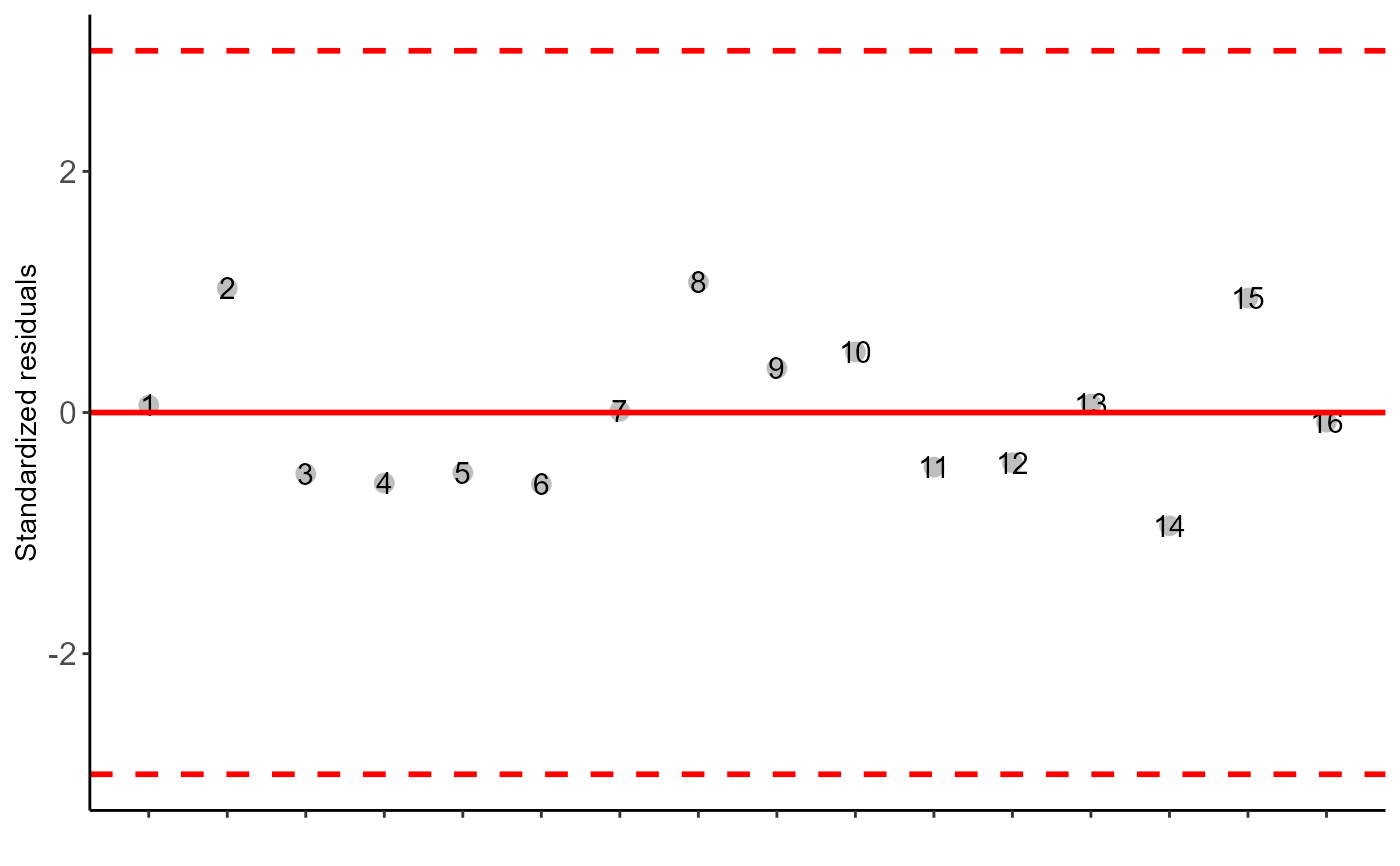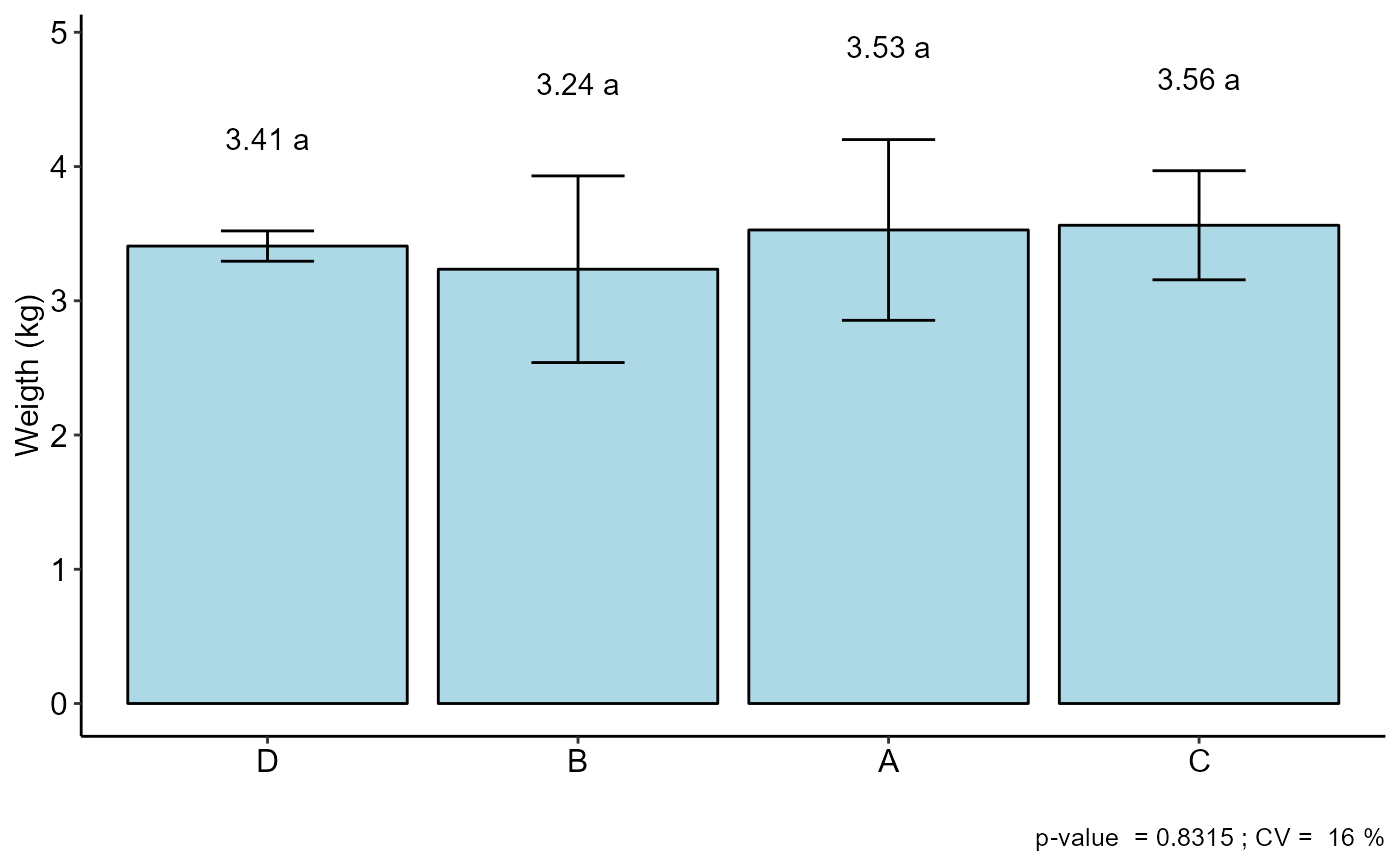This is a function of the AgroR package for statistical analysis of experiments conducted in Latin Square and balanced design with a factor considering the fixed model.

DQL(
trat,
line,
column,
response,
norm = "sw",
homog = "bt",
alpha.f = 0.05,
alpha.t = 0.05,
quali = TRUE,
mcomp = "tukey",
grau = 1,
transf = 1,
constant = 0,
geom = "bar",
theme = theme_classic(),
sup = NA,
CV = TRUE,
ylab = "Response",
xlab = "",
textsize = 12,
labelsize = 4,
fill = "lightblue",
angle = 0,
family = "sans",
dec = 3,
width.column = NULL,
width.bar = 0.3,
errorbar = TRUE,
posi = "top",
point = "mean_sd",
angle.label = 0,
ylim = NA
)

## Arguments

trat

Numerical or complex vector with treatments

line

Numerical or complex vector with lines

column

Numerical or complex vector with columns

response

Numerical vector containing the response of the experiment.

norm

Error normality test (default is Shapiro-Wilk)

homog

Homogeneity test of variances (default is Bartlett)

alpha.f

Level of significance of the F test (default is 0.05)

alpha.t

Significance level of the multiple comparison test (default is 0.05)

quali

Defines whether the factor is quantitative or qualitative (default is qualitative)

mcomp

Multiple comparison test (Tukey (default), LSD, Scott-Knott and Duncan)

grau

Degree of polynomial in case of quantitative factor (default is 1)

transf

Applies data transformation (default is 1; for log consider 0; angular for angular transformation)

constant

Add a constant for transformation (enter value)

geom

Graph type (columns, boxes or segments)

theme

ggplot2 theme (default is theme_classic())

sup

Number of units above the standard deviation or average bar on the graph

CV

Plotting the coefficient of variation and p-value of Anova (default is TRUE)

ylab

Variable response name (Accepts the expression() function)

xlab

Treatments name (Accepts the expression() function)

textsize

Font size

labelsize

Label size

fill

Defines chart color (to generate different colors for different treatments, define fill = "trat")

angle

x-axis scale text rotation

family

Font family

dec

Number of cells

width.column

Width column if geom="bar"

width.bar

Width errorbar

Plot the average value on the graph (default is TRUE)

errorbar

Plot the standard deviation bar on the graph (In the case of a segment and column graph) - default is TRUE

posi

Legend position

point

Defines whether to plot mean ("mean"), mean with standard deviation ("mean_sd" - default) or mean with standard error ("mean_se"). For parametric test it is possible to plot the square root of QMres (mean_qmres).

angle.label

label angle

ylim

Define a numerical sequence referring to the y scale. You can use a vector or the seq command.

## Value

The table of analysis of variance, the test of normality of errors (Shapiro-Wilk ("sw"), Lilliefors ("li"), Anderson-Darling ("ad"), Cramer-von Mises ("cvm"), Pearson ("pearson") and Shapiro-Francia ("sf")), the test of homogeneity of variances (Bartlett ("bt") or Levene ("levene")), the test of independence of Durbin-Watson errors, the test of multiple comparisons (Tukey ("tukey"), LSD ("lsd"), Scott-Knott ("sk") or Duncan ("duncan")) or adjustment of regression models up to grade 3 polynomial, in the case of quantitative treatments. The column, segment or box chart for qualitative treatments is also returned. The function also returns a standardized residual plot.

## Note

The ordering of the graph is according to the sequence in which the factor levels are arranged in the data sheet. The bars of the column and segment graphs are standard deviation.

CV and p-value of the graph indicate coefficient of variation and p-value of the F test of the analysis of variance.

In the final output when transformation (transf argument) is different from 1, the columns resp and respo in the mean test are returned, indicating transformed and non-transformed mean, respectively.

## Author

Gabriel Danilo Shimizu, shimizu@uel.br

Leandro Simoes Azeredo Goncalves

Rodrigo Yudi Palhaci Marubayashi

## Examples

library(AgroR)
data(porco)
with(porco, DQL(trat, linhas, colunas, resp, ylab="Weigth (kg)"))
#>
#> -----------------------------------------------------------------
#> Normality of errors (Shapiro-Wilk
#> -----------------------------------------------------------------
#>                          Method Statistic   p.value
#>  Shapiro-Wilk normality test(W) 0.9183353 0.1585848
#>
#> As the calculated p-value is greater than the 5% significance level, hypothesis H0 is not rejected. Therefore, errors can be considered normal
#>
#> -----------------------------------------------------------------
#> Homogeneity of Variances
#> -----------------------------------------------------------------
#>                               Method Statistic   p.value
#>  Bartlett test(Bartlett's K-squared)  1.207666 0.7511662
#>
#> As the calculated p-value is greater than the 5% significance level, hypothesis H0 is not rejected. Therefore, the variances can be considered homogeneous
#>
#> -----------------------------------------------------------------
#> Independence from errors
#> -----------------------------------------------------------------
#>                  Method Statistic   p.value
#>  Durbin-Watson test(DW)  2.028993 0.2932159
#>
#> As the calculated p-value is greater than the 5% significance level, hypothesis H0 is not rejected. Therefore, errors can be considered independent
#>
#> -----------------------------------------------------------------
#> -----------------------------------------------------------------
#>
#> CV (%) =  16
#> MStrat/MST =  0.1
#> Mean =  3.4331
#> Median =  3.39
#> Possible outliers =  No discrepant point
#>
#> -----------------------------------------------------------------
#> Analysis of Variance
#> -----------------------------------------------------------------
#>           Df    Sum Sq    Mean.Sq   F value     Pr(F)
#> trat       3 0.2622187 0.08740625 0.2898586 0.8315413
#> line       3 0.1065687 0.03552292 0.1178019 0.9463594
#> column     3 1.4274687 0.47582292 1.5779347 0.2899683
#> Residuals  6 1.8092875 0.30154792
#>
#> As the calculated p-value is greater than the 5% significance level, H0 is not rejected
#>
#> -----------------------------------------------------------------
#> Multiple Comparison Test: Tukey HSD
#> -----------------------------------------------------------------
#>  "H0 is not rejected"
#>
#>